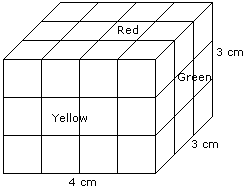# Verbal Reasoning - Cube and Cuboid - Discussion

The following questions are based on the information given below:

1. There is a cuboid whose dimensions are 4 x 3 x 3 cm.
2. The opposite faces of dimensions 4 x 3 are coloured yellow.
3. The opposite faces of other dimensions 4 x 3 are coloured red.
4. The opposite faces of dimensions 3 x 3 are coloured green.
5. Now the cuboid is cut into small cubes of side 1 cm.

2.

How many small cubes have three faces coloured ?

 [A]. 24 [B]. 20 [C]. 16 [D]. 8

Explanation:Such cubes are related to the corners of the cuboid and there are 8 corners.

Hence, the required number is 8.

 Ramsankarbabu said: (Feb 28, 2012) How ? explain!

 Sanju said: (Mar 5, 2017) Anyone explain this.

 An Mishra said: (Jul 12, 2017) Formula= (x-2) 2/corner cube = 8.

 Anusha said: (Sep 5, 2017) Explain this.